CAT  >  Test: XAT Quant 2019

# Test: XAT Quant 2019

Test Description

## 26 Questions MCQ Test Quantitative Aptitude (Quant) | Test: XAT Quant 2019

Test: XAT Quant 2019 for CAT 2022 is part of Quantitative Aptitude (Quant) preparation. The Test: XAT Quant 2019 questions and answers have been prepared according to the CAT exam syllabus.The Test: XAT Quant 2019 MCQs are made for CAT 2022 Exam. Find important definitions, questions, notes, meanings, examples, exercises, MCQs and online tests for Test: XAT Quant 2019 below.
Solutions of Test: XAT Quant 2019 questions in English are available as part of our Quantitative Aptitude (Quant) for CAT & Test: XAT Quant 2019 solutions in Hindi for Quantitative Aptitude (Quant) course. Download more important topics, notes, lectures and mock test series for CAT Exam by signing up for free. Attempt Test: XAT Quant 2019 | 26 questions in 40 minutes | Mock test for CAT preparation | Free important questions MCQ to study Quantitative Aptitude (Quant) for CAT Exam | Download free PDF with solutions
 1 Crore+ students have signed up on EduRev. Have you?
Test: XAT Quant 2019 - Question 1

### For the following questions answer them individuallyA, B, C, D and E are five employees working in a company. In two successive years, each of them got hikes in his salary as follows:A: p% and (p+1)%,B: (p+2)% and (p-1)%,C: (p+3)% and (p-2)%,D: (p+4)% and (p-3)%,E: (p+5)% and (p-4)%.Q. If all of them have the same salary at the end of two years, who got the least hike in his salary?

Detailed Solution for Test: XAT Quant 2019 - Question 1 Let the initial salary of A,B,C,D,E be respectively and let the final salary of everyone be x

Now, a * (1 + p / 100) * (1 + p + 1 / 100) = x

=>a = x / 1 + p / 100) * (1 + p + 1 / 100)

=> a = x * 100 * 100 / (p + 100) * (100 + p + 1)

=> a = x * 100 * 100 / (p + 100) * (p + 101)

Similarly, b = x (1 + p + 2 / 100) * (1 + p – 1 / 100)

=> b = x * 100 * 100 / (p + 102) * (p + 99)

Similarly, c = x / (1 + p + 3 / 100) * (1 + p – 2 / 100)

=> c = x * 100 * 100 / (p + 100) * (p + 98)

Similarly, d = x / (1 + p + 4 / 100) * (1 + p – 3 / 100)

=> d = x * 100 * 100 / (p + 104) * (p + 97)

Similarly, e = x / (1 + p + 5 / 100) * (1 + p – 4 / 100)

=> e = x * 100 * 100 / (p + 105) * (p + 96)

The numerators of the fractions are the same, therefore the one with the smallest value of denominator will have the greatest value. If we compare the denominators, we can find out the fraction with the highest value. The person with the highest initial salary got the least raise, as we know that the final salary of all the candidates is the same.

Thus, denominator of a, aden = (p + 100) ∗ (p + 101) = p2 + 201p + 100 ∗ 101

Similarly, bden = (p + 102) ∗ (p + 99) = p2 + 201p + 102 ∗ 99

cden = (p + 103) ∗ (p + 98) = p2 + 201p + 103 ∗ 98

dden = (p + 104) ∗ (p + 97) = p2 + 201p + 104 ∗ 97

eden = (p + 105) ∗ (p + 96) = p2 + 201p + 105 ∗ 96

We see that we need to compare only the last terms of the denominators as the other terms are the same.

Thus, last term of a, alt = 100 ∗ 101 = 100.52 − 0.52

last term of b, blt = 102 ∗ 99 = 100.52 − 1.52

last term of c, clt = 103 ∗ 98 = 100.52 − 2.52

last term of d, dlt = 104 ∗ 97 = 100.52 − 3.52

last term of e, dlt = 105 ∗ 96 = 100.52 − 4.52

Thus, we can see that since the denominator of is the smallest, therefore E has the highest initial salary.

Test: XAT Quant 2019 - Question 2

### A ﬁrm pays its ﬁve clerks Rs. 15,000 each, three assistants Rs. 40,000 each and its accountant Rs. 66,000. Then the mean salary in the ﬁrm comprising of these nine employees exceeds its median salary by rupees

Detailed Solution for Test: XAT Quant 2019 - Question 2 There are 9 employees in the firm. Median of any set is the element which occurs in the middle of the set when the elements are positioned in increasing or decreasing order. The number of elements in this set = 5+3+1 = 9

Thus, the middle element will be in the position (9 + 1)/2= 5th. Since we know that the clerks are paid the least, the clerk salaries would be in the beginning of the set if arranged in ascending order. Thus, the 5th element will be a clerk's salary as the number of clerks is 5.

Thus, median of the set = Rs 15,000.

To find the mean salary, we first need to find the sum total salary of the people involved.

Total salary = (Number of clerks*salary of 1 clerk) + (Number of assistants*salary of 1 assistant) + (Number of accountants*salary of 1 accountant)

⇒ Total salary = (5*15,000)+(3*40,000)+(1*66,000)

⇒ Total salary = 75,000+1,20,000+66,000

⇒ Total salary = 2,61,000

Mean salary = Total Salary/Total number of employees

⇒ Mean salary = 261000/9

⇒ Mean salary = Rs 29,000/-

Thus, difference between mean and median salary = 29,000-15,000 = Rs 14,000

Test: XAT Quant 2019 - Question 3

### Two numbers a and b are inversely proportional to each other. If a increases by 100%, then b decreases by:

Detailed Solution for Test: XAT Quant 2019 - Question 3 Inverse proportionality is expressed as follows

a ∝ 1/b

⇒ a*b = constant

When the value of a changes, the value of b changes accordingly such that their product remains the same.

Thus, new value of a α = α + α = 2α

Thus, the new value of b b’ can be solved by:

α’ * b’ = α * b

⇒ 2α ∗ b’ = α ∗ b

⇒ b’ = b/2

Thus, b decreases by 50%.

Test: XAT Quant 2019 - Question 4

If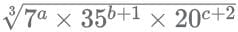is a whole number then which one of the statements below is consistent with it?

Detailed Solution for Test: XAT Quant 2019 - Question 4 In the given statement, the expression becomes a whole number only when the powers of all the prime numbers are also whole numbers.

Let us first simplify the expression a bit by expressing all terms in terms of prime numbers.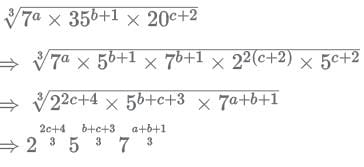Now, from the given options, we can put in values of the variables and check the exponents of all the numbers.

Option A: a = 2, b = 1, c = 1:

In this case, we can see that the exponent of 5 i.e. (b+c+3)/3 = 5/3 is not a whole number.

Option B: a = 1, b = 2, c = 2

In this case, we can see that the exponent of 2 i.e. (2c+4)/3 = 8/3 is not a whole number.

Option C: a = 2, b = 1, c = 2

In this case, we can see that the exponent of 2 i.e. (2c+4)/3 = 8/3 is not a whole number.

Option D: a = 3, b = 1, c = 1

In this case, we can see that the exponent of 5 i.e. (b+c+3)/3 = 5/3 is not a whole number.

Option E: a = 3, b = 2, c = 1

In this case, we can see that all exponents are whole numbers. Thus, option E is the correct option.

Test: XAT Quant 2019 - Question 5

A gold ingot in the shape of a cylinder is melted and the resulting molten metal molded into a few identical conical ingots. If the height of each cone is half the height of the original cylinder and the area of the circular base of each cone is one ﬁfth that of the circular base of the cylinder, then how many conical ingots can be made?

Detailed Solution for Test: XAT Quant 2019 - Question 5 Let the radius of the cylinder be and height be (as shown).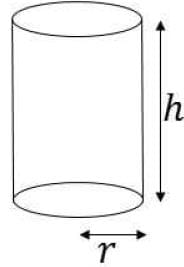Let the dimensions of the cone be and (as shown).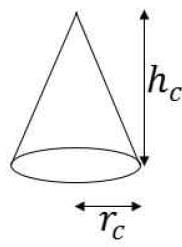It is given that hc = h/2

Area of base of cone Ac = π × r2c

Area of base of cylinder A = π × r2

Given, Ac = 1/5 × A

⇒π x r2c = 1 / 5 x π x r2

⇒ r2 / r2c = 5

Now we know that the volume of cylinder = total volume of cones

Let the number of cones be n.

So, volume of cylinder = n x volume of each cone

⇒ V = n × Vc

⇒ π × r2 × h = n × 1/3 × π × r2c × hc

⇒ r2 × h = n × 1/3 × r2c× hc

⇒ r2 / r2c x h / hc x 3 = n

⇒ 5 × 2 × 3 = n

⇒ 30 = n

Thus, 30 conical ingots can be made.

Test: XAT Quant 2019 - Question 6

An article is marked x% above the cost price. A discount of 2/3x% is given on the marked price. If the profit is 4% of the cost price and the value of x lies between 25 and 50, then the value of 50% of x is?

Detailed Solution for Test: XAT Quant 2019 - Question 6 Let CP of the object be a.

It is given that SP = (1 + 4/100) x CP

⇒ SP = 1.04 x CP

It is given that MP = (1 + x / 100) x CP

It is also given that SP = (1 – 2x / 3 / 100) x MP

⇒ SP = (1 – 2x / 300) x (1 + x / 100) x CP

⇒ (1 + 4 / 100) x CP = (1 –2x / 300) x (1 + x / 100) x CP

⇒(1 + 4 / 100) = (1 –2x / 300) x (1 + x / 100)

⇒(1 + 4 / 100) = (1 – 2x / 300) x (1 + x / 100)

⇒104 / 100 = (1 – 2x / 300) x (1 + x / 100)

⇒104 / 100 = (300 – 2x / 300) x (x + 100 / 100)

⇒ 104/100 × 300 × 100 = (300 − 2x) x (x + 100)

⇒ 31200 = (300 − 2x) x (x + 100)

Now, we look at the options.

Since the question says that x∈[25,30] so 50% of x i.e. 0.5x cannot be less than 12.50 ie 25/2 and cannot be more than 25 i.e. 50/2. This eliminates option A.

Putting values of given in the remaining options in the final expression, [here we need to be careful to use the value of x and not the value of 50% of x as given in the expression]

Look carefully in the remaining options: 16,13,15 as 0.5x i.e., 32,26,30 as possible values of x.

In the question since the discount rate offered is %, then a safer choice would be to check for the option of 30 in the beginning. It is a safer choice because the percentage of discount that we get in the other options are not whole numbers.

NOTE: THIS IS JUST A SAFE CHOICE AND NEVER MARK AN ANSWER DIRECTLY ON THIS PRESUMPTION WITHOUT CHECKING IT.

Putting x=30 in the expression:

(300 - (2x30))x(30+100) = (300 – 60)x(100+30) = 240x130 = 24x13x100= 312x100 = 31200= LHS of expression.

Thus the value of is 30.

Therefore, value of 50% of = 0.5x30 = 15

Test: XAT Quant 2019 - Question 7

A computer is sold either for Rs.19200 cash or for Rs.4800 cash down payment together with five equal monthly instalments. If the rate of interest charged is 12% per annum, then the amount of each instalment (nearest to a rupee) is:

Detailed Solution for Test: XAT Quant 2019 - Question 7 Amount on which interest will be charged = 19200 - 4800 = 14400

The total amount paid will be equal to the sum of all monthly instalments. Therefore, we have

14400 ∗ k5a = I (k4a + k3a + k2a + ka + 1) ....(1)

where, k = 1 + 12/100 & a = 1/12

We know that, k5a − 1 = (k − 1)(k4a + k3a + k2a + ka + 1)

⇒ k4a + k3a + k2a + ka + 1 k-1/k-1 ...(2)

Substituting in equation (1) we get

I = 14400 ∗ k∗ [k−1/k−1] ...(3)

On substituting the values of k and a in equation (3) we get

I ≈ 2965

Hence, option B.

Test: XAT Quant 2019 - Question 8

When opening his fruit shop for the day a shopkeeper found that his stock of apples could be perfectly arranged in a complete triangular array: that is, every row with one apple more than the row immediately above, going all the way up ending with a single apple at the top.

During any sales transaction, apples are always picked from the uppermost row, and going below only when that row is exhausted.

When one customer walked in the middle of the day she found an incomplete array in display having 126 apples totally. How many rows of apples (complete and incomplete) were seen by this customer? (Assume that the initial stock did not exceed 150 apples.)

Detailed Solution for Test: XAT Quant 2019 - Question 8 The stack of apples can be imagined as shown below. For every row, there is an increase of 1 apple from the previous row.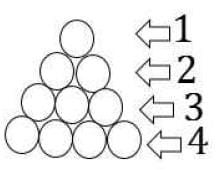This means that the sum total of the apples present in the stack will be the sum of AP given as : 1,2,3,4.... i.e. the sum of numbers from 1.

This is given by the formula S = (n×(n+1))/2

Since the initial number of apples was not more than 150, we find the maximum number of rows that was possible.

Let S be the total initial sum of apples in the cart.

We know that S ≤ 150

⇒ n×(n+1)/2 ≤ 150

⇒ n x (n + 1) ≤ 300

⇒ n2 + n ≤ 300

Since the biggest number with a square less than 300 is 17, we try to see if 17 works in the expression by hit and trial method.

So, 17 x 18 = 306 > 300, therefore the maximum number of rows possible is not 17.

Since 306 is JUST over 300, let us check at 16.

So, 16 x 17 = 272 < 300,="" therefore="" the="" maximum="" number="" of="" rows="" possible="" is="" />

Let us tabulate the total number of apples present in the cart based on the number of rows: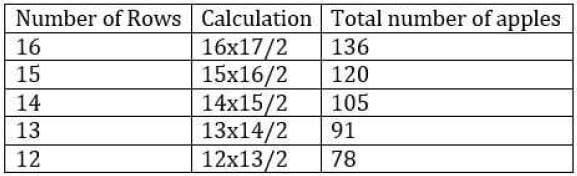Since the total number of apples from row 15 is less than 126 (which was found by the customer), we can safely say that the total number of rows of apples with the shopkeeper that day was 16.

Now, if 'n' rows of apples are sold, the total available apples can be calculated as:

16 x 17 / 2 – n x (n + 1) / 2

Let us tabulate the result obtained in this case: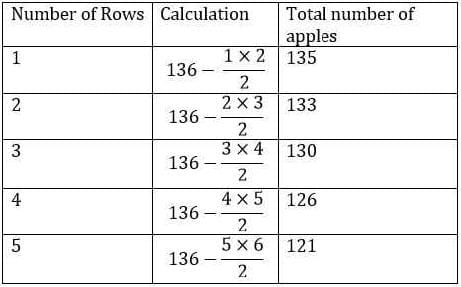From the table we can see that when the customer saw 126 apples, the apples in the top 4 rows had been sold.

Therefore, the number of rows = 16 - 4 = 12 rows.

Test: XAT Quant 2019 - Question 9

Let P be the point of intersection of the lines 3x + 4y = 2a and 7x + 2y = 2018 and Q the point of intersection of the lines 3x + 4y = 2018 and 5x + 3y = 1. If the line through P and Q has slope 2, the value of a is:

Detailed Solution for Test: XAT Quant 2019 - Question 9 On solving for x and y from the equations

3x + 4y = 2018 and 5x + 3y = 1

we get Q(-550,917)

Let, P(x, y)

So, y – 917/x + 550 = 2

⇒ y - 2x = 2017 ...(1)

Considering the equations

3x + 4y = 2a ........(2)

7x + 2y = 2018 .....(3)

On subtracting equation (2) from (3) we have,

4x - 2y = 2018 - 2a

=> 2x - y = 1009 - a

=> y - 2x = a -1009 .....(4)

From equation (1) and (4)

2017 = a - 1009

=> a = 3026

Hence, option C.

Test: XAT Quant 2019 - Question 10

Let C be a circle of radius √20 cm. Let L1, L2 be the lines given by 2x − y −1 = 0 and x + 2y−18 = 0, respectively. Suppose that L1 passes through the center of C and that L2 is tangent to C at the point of intersection of L1 and L2. If (a, b) is the center of C, which of the following is a possible value of a + b?

Detailed Solution for Test: XAT Quant 2019 - Question 10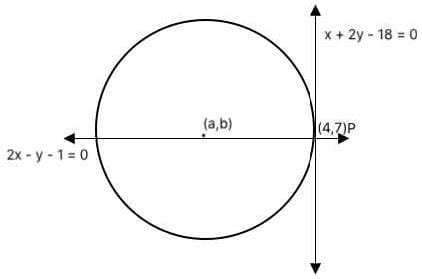As mentioned in the question,

Lines L1 and L2 intersect at point P as shown in the figure.

On solving for x and y from equations

x + 2y - 18 = 0

2x - y - 1 = 0

We get x = 4 and y =7.

Given, radius = √20

Using the equation of a circle, we have

(4 − a)2 (7 − b)2 = 20....(1)

The center of the circle will lie on the line: 2x - y - 1 = 0

a,b will satisfy this equation.

So 2a – b-1=0

B=2a-1

From equation 1…

(4 − a)2 (7 − b)2 = 20

(4 − a)2 (8 − 2a)2 = 20

5(4 − a)2 = 20

a = 6 or a = 2

b = 11 or b = 3

The sum of the coordinates possible = 6+11 or 2+3

i.e. 17 or 5

Option B is one of the solutions.

Test: XAT Quant 2019 - Question 11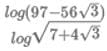equals which of the following?

Detailed Solution for Test: XAT Quant 2019 - Question 11 Simplify the expression a bit to remove the root sign in the denominator

1log 97 − 56√3

2 × (log7 + 4√3)

=> 2 x log 97 - 56√3 / log7 + 4√3

To move further, let us see the root of the numerator.

Assume the root of the numerator to be √a - √b.

When we square it, we get, a + b − (2 × √a√b) = a + b − 2√ab

comparing the value of terms under root with the terms in the numerator, we get

√ab = 28√3 and a + b = 97

from solving this, we get to know that and α = 7 and b = 4√3

log 7 − 4√3

Thus, the expression can be written as 2 × 2 × log 7 + 4√3

=> 4 x log 7 - 4√3 / log7 + 4√3

Now, let us look at the reciprocal of the term in log in the denominator.

1 / 7 + 4√3 = 1 / 7 + 4√3 x 7 - 4√3 / 7 - 4√3

=> 7 - 4√3 / 72 – (4√3)2

=> 7 - 4√3 / 49 – 48 = 7 - 4√3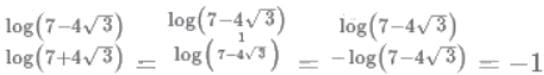Thus the value of the expression can be further simplified as

⇒ 4 × (−1) = −4

Hence the correct answer is option C.

Test: XAT Quant 2019 - Question 12

In the trapezium ABCD the sides AB and CD are parallel. The value ofsin∠BAC / sin∠BAD is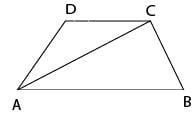Detailed Solution for Test: XAT Quant 2019 - Question 12 Construct a perpendicular in the trapezium. Let its height be 'h'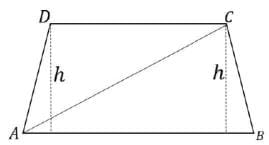Now, sin∠BAC = h/AC

Thus, sin∠BAC /sin∠BAD = h / AC / h / AD = AD / AC

Test: XAT Quant 2019 - Question 13

In the picture below, EFGH, ABCD are squares, and ABE, BCF, CDG, DAH are equilateral triangles. What is the ratio of the area of the square EFGH to that of ABCD?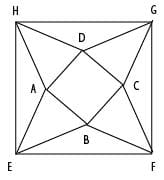Detailed Solution for Test: XAT Quant 2019 - Question 13 Let the sides of the smaller square be units and the sides of the larger square be units.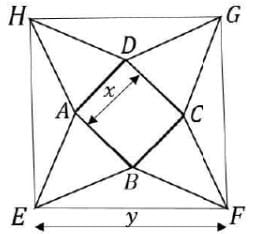Construct diagonal HF on the larger square. Let the point of intersection of AD and diagonal be I and point of intersection of BC and diagonal be J.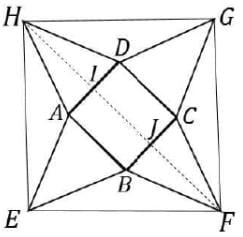We know that since EFGH is a square, the diagonal will bisect the angle. Therefore ∠EHF = 45o

Observe ΔAEH and ΔDHG:

AE=AH=DH=DG = x units and EH=HG= y units.

Thus, by SSS property, ΔAEH ≅ ΔDHG

Thus, we know that ∠AHE= ∠DHG

Also, ∠AHE+ ∠DHG+ ∠AHD= 90o (angle of a square)

⇒ (2 × ∠AHE) + 60o = 90o (angle of an equilateral triangle)

⇒ (2 × ∠AHE) = 30o

⇒∠AHE = 15o

Since ∠AHE = 15o, ∠AHI = 45o − 15o = 30o

Since 30o = 2 we can say that HF is the angle bisector of ∠AHD

Since ΔAHD is an equilateral triangle, we know that the angle bisector, median and altitude will all be the same line i.e. HI in this case.

By symmetry, we know that JF will also be the angle bisector, median and altitude.

Also, length of altitude of equilateral triangle i.e. HI and JF = (√3 x x)/2

Length of diagonal HF = √2 x y

From the figure, we can express the length of diagonal HF as given:

√2 x y = √3 x x / 2 + x + √3 x x / 2

=>√2 x y = (√3 x x) + x

=>√2 x y = (√3 + 1) + x

=>√2 x y = x x (√3 + 1)

=> y = x x (√3 + 1 / √2)

Ratio of areas asked = y / (x)2 = √3 + 1 / (√2)2 = 4 + 2√3 / 2 = 2 + √3

Test: XAT Quant 2019 - Question 14

What is the maximum number of points that can be placed on a circular disk of radius 1 metre (some of the points could be placed on the bounding circle of the disk) such that no two points are at a distance of less than 1 metre from each other?

Detailed Solution for Test: XAT Quant 2019 - Question 14 In order to accommodate a maximum number of points on the circular disk, all the points should be placed on the boundary of the disk as any point if placed inside the boundary would be less than 1 metre apart from the centre of the disk. Also, all the points have to be exactly 1 metre apart from each other to place a maximum number of points. Hence, a maximum of 6 points can be placed on the boundary of the disk (each adjacent point placed 60 degrees apart) and 1 point at the centre.

Hence, a total of 7 points can be placed on the disk.

Test: XAT Quant 2019 - Question 15

The figure below shows two right angled triangles ∆OAB and ∆OQP with right angles at vertex A and P, respectively, having the common vertex O, The lengths of some of the sides are indicated in the figure. (Note that the figure is not drawn to scale.) AB and OP are parallel.

What is ∠QOB?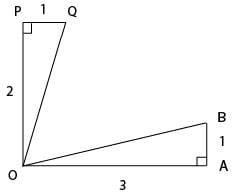Detailed Solution for Test: XAT Quant 2019 - Question 15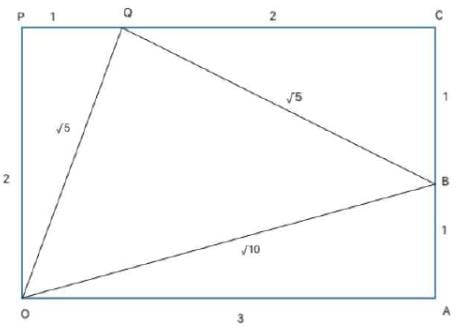The figure can be redrawn as shown above.

∵ AB and OP are parallel, POAC is a rectangle.

In right △QCB, QB = ∠5

△QBO is an isosceles right-angled triangle with ∠OQB = 90° (By Pythagoras theorem)

∴∠QOB = 45°

Test: XAT Quant 2019 - Question 16

Consider the function f(x) = (x + 4)(x + 6)(x + 8) ⋯ (x + 98). The number of integers x for which f(x) < 0 is:

Detailed Solution for Test: XAT Quant 2019 - Question 16 The critical points of the function are -4, -6, -8, ... , -98 (48 points).

For all integers less than -98 and greater than -4 f(x) > 0 always.

for x = -5, f(x) < 0

Similarly, for x= -9, -13, ...., -97 (This is an AP with common difference -4)

Hence, in total there are 24 such integers satisfying f(x)< 0.

Test: XAT Quant 2019 - Question 17

Let ABC be an isosceles triangle. Suppose that the sides AB and AC are equal and let the length of AB be x cm. Let b denote the angle ∠ABC and sin b = 3/5. If the area of the triangle ABC is M square cm, then which of the following is true about M?

Detailed Solution for Test: XAT Quant 2019 - Question 17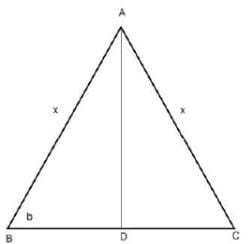ABC can be represented as shown above where AD ⊥ BC

Given, Sin b = 3/5

Hence, AD = 3x/5 and BD = 4x/5

Now, BC = 2 BD = 8x/5

∴ area of △ABC = 1/2 * BC* AD = 1/2 *  8x/5 * 3x/5 = 12x2/25

Only option E satisfies.

Hence, option E.

Test: XAT Quant 2019 - Question 18

If x2 + x + 1 = 0, then x2018 + x2019 equals which of the following:

Detailed Solution for Test: XAT Quant 2019 - Question 18 We know that,

x3 − 1 = (x − 1)(x2 + x + 1)

Since, x2 + x + 1 = 0

∴ x3 − 1 = 0

⇒ x3 = 1

Now, x2018 + x2019

= (x3)672∗ x2 (x3)673

= 1672∗ x2 + 1673

= x2 + 1

= -x

Hence, option C.

Test: XAT Quant 2019 - Question 19

We have two unknown positive integers m and n, whose product is less than 100.

There are two additional statements of facts available:

mn is divisible by six consecutive integers { j, j + 1,...,j + 5 }

m + n is a perfect square.

Q. Which of the two statements above, alone or in combination shall be sufficient to determine the numbers m and n?

Detailed Solution for Test: XAT Quant 2019 - Question 19 Given, m and n are two positive integers having product less than 100

Statement 1:

mn is divisible by 6 consecutive integers. This means mn must be divisible by the LCM of these 6 integers multiples.

Only numbers from 1 to 6 satisfy this with LCM 60. Any other set of 6 consecutive integers clearly exceeds 100 as its LCM.

Only such a number satisfying the condition is 60.

60 can further be expressed as the product of two positive integers in the following ways:

1 x 60, 2 x 30, 3 x 20, 4 x 15, 5 x 12 and 6 x 10.

So, no unique values of m and n can be determined from the above.

Statement 2:

m + n is a perfect square.

From this statement alone too no unique set of solutions can be determined.

From Statements 1 and 2: Only the pair 6 x 10 satisfies the condition.

Hence, both statements taken together are suﬃcient to answer the question, but neither statement alone is suﬃcient.

Test: XAT Quant 2019 - Question 20

A bag contains marbles of three colours-red, blue and green. There are 8 blue marbles in the bag.

There are two additional statements of facts available: If we pull out marbles from the bag at random, to guarantee that we have at least 3 green marbles, we need to extract 17 marbles.

If we pull out marbles from the bag at random, to guarantee that we have at least 2 red marbles, we need to extract 19 marbles.

Q. Which of the two statements above, alone or in combination shall be sufficient to answer the question "how many green marbles are there in the bag"?

Detailed Solution for Test: XAT Quant 2019 - Question 20 From statement 1

∴ Red + Blue + Green = 17

Since, this ensures that there are at least 3 green marbles, so remaining marbles must be red and blue.

Red + Blue = 17 - 3 = 14

Blue = 14 - Red = 14 - 8 = 6

But this doesn't give us the total number of green marbles. So, statement 1 alone is not sufficient.

From statement 2:

Red + Blue + Green = 19

Since, this ensures that there are at least 2 red marbles, so remaining marbles must be blue and green

∴ Blue + Green = 19 - 2 = 17

Again, Green = 17 - Blue = 17 - 8 = 9.

Hence, statement 2 alone is sufficient to answer the question.

Test: XAT Quant 2019 - Question 21

The break-up of the students in a university by subject major is given in the polar pie-chart. The bar chart shows the number of students who major in physics by geographic location.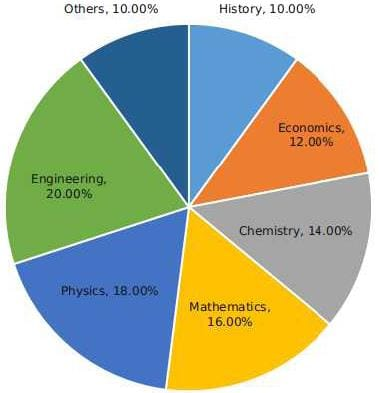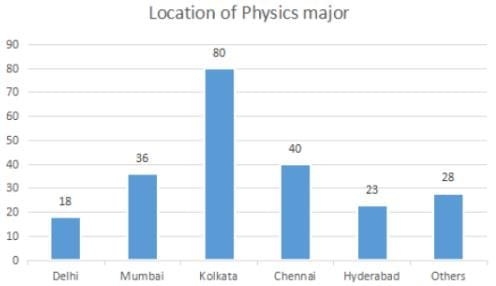Q. How many students major in chemistry?

Detailed Solution for Test: XAT Quant 2019 - Question 21 Total number of Physics major from all locations = 225

∴ Total number of students = 225/0.18 = 1250

Number of students majoring in chemistry = 14% * 1250 = 175

Hence, option B.

Test: XAT Quant 2019 - Question 22

The break-up of the students in a university by subject major is given in the polar pie-chart. The bar chart shows the number of students who major in physics by geographic location.Q. If the proportion of physics majors who are from Delhi is the same as the proportion of engineering majors who are from Delhi, how many engineering majors are from Delhi?

Detailed Solution for Test: XAT Quant 2019 - Question 22 Total number of Physics major from all locations = 225

∴ Total number of students = 225/0.18 = 1250

Number of engineering students = 20% * 1250 = 250

So, basis the given condition we have

⇒ 18/225 = x/250

⇒ x = 20

Hence, option D

(Note: This question has been presented as was in the actual paper and has been solved by the aid of visual inference)

Test: XAT Quant 2019 - Question 23

The break-up of the students in a university by subject major is given in the polar pie-chart. The bar chart shows the number of students who major in physics by geographic location.Q. 12% of all students are from Chennai. What is the largest possible percentage of economics students that can be from Chennai, rounded oﬀ to the nearest integer?

Detailed Solution for Test: XAT Quant 2019 - Question 23 Total number of Physics major from all locations = 225

∴ Total number of students = 225/0.18 = 1250

Students from Chennai = 12% * 1250 = 150

Given, number of Physics majors from chennai = 40

∴ maximum possible number of economic students from chennai = 150 - 40 = 110

Maximum percentage of students = 110/150 % = 73.33 %

Hence, option A.

Test: XAT Quant 2019 - Question 24

Given below is the time table for a trans-continental train that cuts across several time zones. All timings are in local time in the respective cities. The average speed of the train between any two cities is the same in both directions.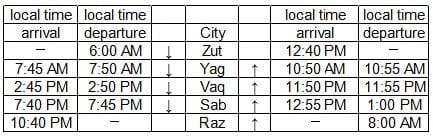Q. Which of the following pairs of cities are in the same time zone?

Detailed Solution for Test: XAT Quant 2019 - Question 24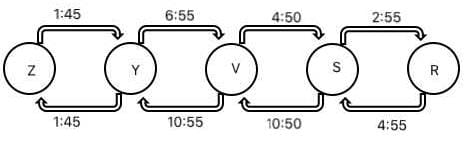The above figure shows the time taken in hrs:min to travel between two consecutive cities both for upward and downward journeys.

The difference in time between two consecutive cities will be the average of the difference in time taken for upward and downward journeys.

So, the difference of time for different consecutive pairs can be found as below:

Z-Y:

For both up & down motions time difference is 0. Hence, Z & Y are in the same time zone.

Y-V:

Time taken for travelling from Y to V is less than that of from V to Y with a difference of 4 hrs. Hence, Y is 2 hours ahead of V.

V-S:

Time taken for travelling from V to S is less than that of from S to V with a difference of 6 hrs. Hence, V is 3 hours ahead of S.

S-R:

Time taken for travelling from S to R is less than that of from R to S with a difference of 2 hrs. Hence, S is 1 hour ahead of R.

As shown above, only cities Zut and Yag are in the same time-zone.

Hence, option C.

Test: XAT Quant 2019 - Question 25

Given below is the time table for a trans-continental train that cuts across several time zones. All timings are in local time in the respective cities. The average speed of the train between any two cities is the same in both directions.Q. What is the total time taken in minutes by the train to go from Zut to Raz?

Detailed Solution for Test: XAT Quant 2019 - Question 25The above figure shows the time taken in hrs:min to travel between two consecutive cities both for upward and downward journeys.

The difference in time between two consecutive cities will be the average of the difference in time taken for upward and downward journeys.

So, the difference of time for different consecutive pairs can be found as below:

Z-Y:

For both up & down motions time difference is 0. Hence, Z & Y are in the same time zone.

Y-V:

Time taken for travelling from Y to V is less than that of from V to Y with a difference of 4 hrs. Hence, Y is 2 hours ahead of V.

V-S:

Time taken for travelling from V to S is less than that of from S to V with a difference of 6 hrs. Hence, V is 3 hours ahead of S.

S-R:

Time taken for travelling from S to R is less than that of from R to S with a difference of 2 hrs. Hence, S is 1 hour ahead of R.

Zut is 6 hours ahead of Rag. Also, the train departs from Zut at 6:00 am and arrives at Rag at 10:40 pm taking a total of 16 hrs 40 min.

Hence, the total time taken to travel from Zut to Rag is 22 hrs 40 min. Hence, option A.

Test: XAT Quant 2019 - Question 26

Given below is the time table for a trans-continental train that cuts across several time zones. All timings are in local time in the respective cities. The average speed of the train between any two cities is the same in both directions.Q. What time is it at Yag when it is 12:00 noon at Sab?

Detailed Solution for Test: XAT Quant 2019 - Question 26The above figure shows the time taken in hrs:min to travel between two consecutive cities both for upward and downward journeys.

The difference in time between two consecutive cities will be the average of the difference in time taken for upward and downward journeys.

So, the difference of time for different consecutive pairs can be found as below:

Z-Y:

For both up & down motions time difference is 0. Hence, Z & Y are in the same time zone.

Y-V:

Time taken for travelling from Y to V is less than that of from V to Y with a difference of 4 hrs. Hence, Y is 2 hours ahead of V.

V-S:

Time taken for travelling from V to S is less than that of from S to V with a difference of 6 hrs. Hence, V is 3 hours ahead of S.

S-R:

Time taken for travelling from S to R is less than that of from R to S with a difference of 2 hrs. Hence, S is 1 hour ahead of R.

It can be concluded that Yag is 5 hours ahead of Sab. Hence, it will be 5:00 Pm at Yag when it is 12 noon at Sab.

Hence, option A.

## Quantitative Aptitude (Quant)

163 videos|152 docs|131 tests
 Use Code STAYHOME200 and get INR 200 additional OFF Use Coupon Code
Information about Test: XAT Quant 2019 Page
In this test you can find the Exam questions for Test: XAT Quant 2019 solved & explained in the simplest way possible. Besides giving Questions and answers for Test: XAT Quant 2019, EduRev gives you an ample number of Online tests for practice

## Quantitative Aptitude (Quant)

163 videos|152 docs|131 tests

### How to Prepare for CAT

Read our guide to prepare for CAT which is created by Toppers & the best Teachers